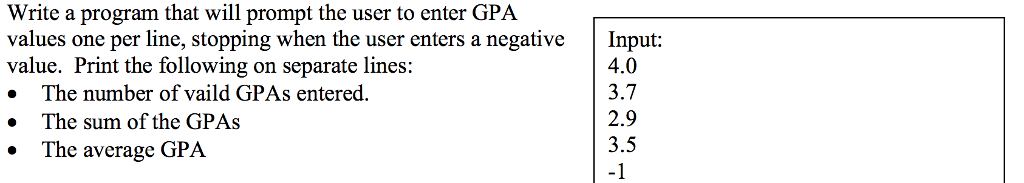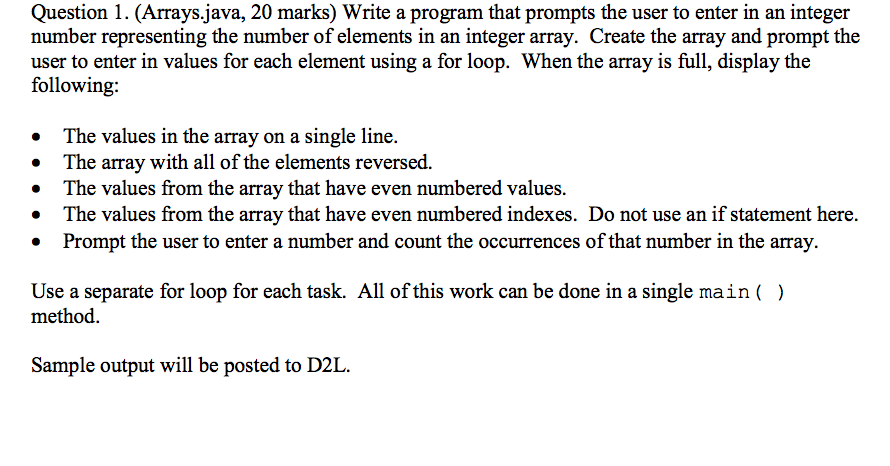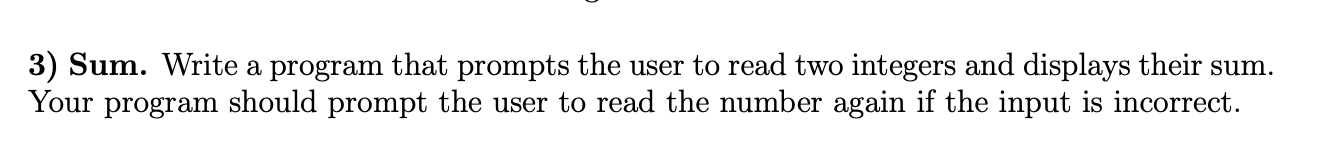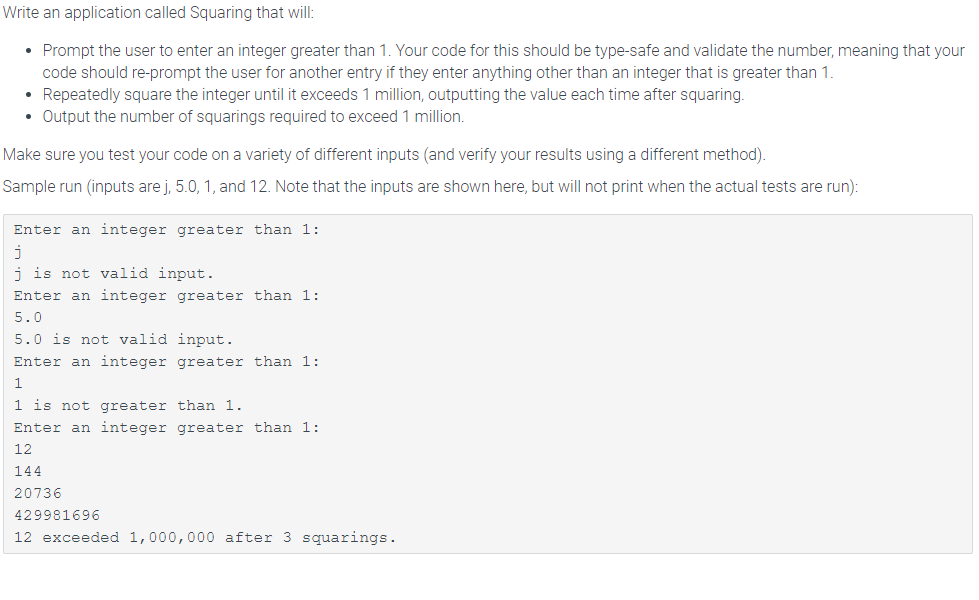Question

Java

Write a program that prompt the user to enter a decimal number representing the radius of a circle. If the number is greater or equal to zero, the program displays the area of the circle. Otherwise, the program displays “negative input”

import java.util.Scanner;

public static void main(String[] args) {

Scanner scan = new Scanner(System.in);

System.out.println("Enter the radius of a circle: ");

System.out.println("Circle Area: "+area);

} else {

System.out.println("negative input");

}

}

}

Output:

Enter the radius of a circle:
8
Circle Area: 201.06192982974676

Enter the radius of a circle:
-1
negative input

#### Earn Coins

Coins can be redeemed for fabulous gifts.

Similar Homework Help Questions
• ### In Java please: Write a program that will prompt the user to enter GPA values one...In Java please: Write a program that will prompt the user to enter GPA values one per line, stopping when the user enters a negative Input: value. Print the following on separate lines: . The number of vaild GPAs entered » The sum of the GPAs 4.0 3.7 2.9 3.5 -1 The average GPA

• ### java programe Write a program that prompts the user to enter in an integer number representing...java programe Write a program that prompts the user to enter in an integer number representing the number of elements in an integer array. Create the array and prompt the user to enter in values for each element using a for loop. When the array is full, display the following: The values in the array on a single line. The array with all of the elements reversed. The values from the array that have even numbered values. The values from...

• ### In Java - Write a program that prompts the user to enter two integers and displays...

In Java - Write a program that prompts the user to enter two integers and displays their sum. If the input is incorrect, prompt the user again. This means that you will have a try-catch inside a loop.

• ### Please write in JAVA ONLY Please use a dialog box to prompt the user to enter...

Please write in JAVA ONLY Please use a dialog box to prompt the user to enter the radius for a circle (radius should be a double). Then, use a Message Box to display the Radius, Area and Circumference of the circle with that Radius. You will use the Math class constant Math.PI to calculate the Area and Circumference.

• ### Write a program that asks the user to enter number, and displays all the numbers that...

Write a program that asks the user to enter number, and displays all the numbers that are multiples of 2 and 5 smaller than or equal to the number entered by the user. Hint: A number n is a multiple of 2 if the remainder of the division of n by 2 is equal to zero. Your program should have an output similar to the following: Please enter a number: 50 The multiples of 2 and 5 less than or...

• ### − Write a C# program that can calculate the area of a circle. − The user will enter the radius of a circle. − Your program should then calculate the area and show the result. − Your program should interact with the user in a decent manner • such as prom

- Write a C# program that can calculate the area of a circle.- The user will enter the radius of a circle.- Your program should then calculate the area and show the result.- Your program should interact with the user in a decent mannersuch as prompt the user to enter radiusand explain the result to user- The formula is given below:area=radius * radius * pie (3.14159)

• ### in java 3) Sum. Write a program that prompts the user to read two integers and...in java 3) Sum. Write a program that prompts the user to read two integers and displays their sum. Your program should prompt the user to read the number again if the input is incorrect.

• ### Write a java program to prompt the user to enter three doubles and compute the sum...

Write a java program to prompt the user to enter three doubles and compute the sum and product. Print "The sum is 999 and the product is 999.", where 999 is replaced with the user sum and product.

• ### in java Write an application called Squaring that will: • Prompt the user to enter an...in java Write an application called Squaring that will: • Prompt the user to enter an integer greater than 1. Your code for this should be type-safe and validate the number, meaning that your code should re-prompt the user for another entry if they enter anything other than an integer that is greater than 1. • Repeatedly square the integer until it exceeds 1 million, outputting the value each time after squaring. • Output the number of squarings required to...

• ### Write a java program that compute the circumference and area of a circle from the user...

Write a java program that compute the circumference and area of a circle from the user input for a radius.